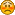###Author Topic: setting 0 value on object rotation  (Read 2528 times)

####.jayderyu

• long
•• Posts: 116##### setting 0 value on object rotation
« on: February 23, 2009, 08:51:20 am »
I'm not sure how to do this.

I load my 3ds file, but the model loads at a "wrong" angle. I don't have the skill to know what the hell i'm doing in an edit.
What i'm trying to do is rotate the object so it's along the angle I want it. Then set the current values as new 0.

Why. I'm trying to add a camera satellite, but when I do the orientation of the model is so off it's really hard to figure out where to put, rotate the satellite for the camera. So if I can get the objected rotated the right way then add the satellite using 0,0,0 as the starting refernce then it would make my life so much easier.

If I knew more about modeling I would probably model in a target and cam location.

I tried the .setRotationMatrix(new Matrix())
but that just put's it back to the original rotational.

####paulscode

• double
••• Posts: 863##### Re: setting 0 value on object rotation
« Reply #1 on: February 23, 2009, 09:58:07 am »
If your .3ds model was exported from 3DS Max, I know the following will work to correct the orientation.  You would do this immediately after loading your object.  This may work for .3ds files exported from other modeling programs as well (I am pretty sure it works for .3ds files exported by Google Sketchup as well).  If not, you might have to play around with the angles to get your model in the correct orientation:

Code: [Select]
`        newObject.rotateX( (float) Math.PI / 2 );        newObject.rotateY( (float) Math.PI );        newObject.rotateZ( (float) Math.PI );        newObject.rotateMesh();        newObject.setRotationMatrix( new Matrix() );`
The above assumes there is only one sub-object in the model, or that the objects were all merged into one.  If you are loading your model with the "children of a dummy-object" method, then you would use this on the array returned by the Loader class:
Code: [Select]
`        for( int x = 0; x < objs.length; x++ )        {            objs[x].rotateX( (float) Math.PI / 2 );            objs[x].rotateY( (float) Math.PI );            objs[x].rotateZ( (float) Math.PI );            objs[x].rotateMesh();            objs[x].setRotationMatrix( new Matrix() );        }`
If this doesn't work, let me know what modeling program you are using, and I'll do a little research to see if I can figure out what angle changes you need to make.

####paulscode

• double
••• Posts: 863##### Re: setting 0 value on object rotation
« Reply #2 on: February 23, 2009, 10:27:41 am »
Opps, sorry - I think I gave you the angles for my "view .3ds" loader, which places the object so it is facing the camera (along the -z axis).  I think you'll want to remove that rotateY, so the object will face into the screen instead (along the +z axis).  This would be equivalent to a Camera's initial orientation.

Code: [Select]
`        newObject.rotateX( (float) Math.PI / 2 );        newObject.rotateZ( (float) Math.PI );        newObject.rotateMesh();        newObject.setRotationMatrix( new Matrix() );`
Sorry about that!####.jayderyu

• long
•• Posts: 116##### Re: setting 0 value on object rotation
« Reply #3 on: February 23, 2009, 10:46:06 am »
thanks you so much. I never thought about rotating the objects instead of the dummy.
Code: [Select]
`      list[i].rotateX( (float) Math.PI / 2 );      list[i].rotateY( (float) Math.PI / 2);      list[i].rotateZ( (float) Math.PI );`
This got me exactly what I wanted.